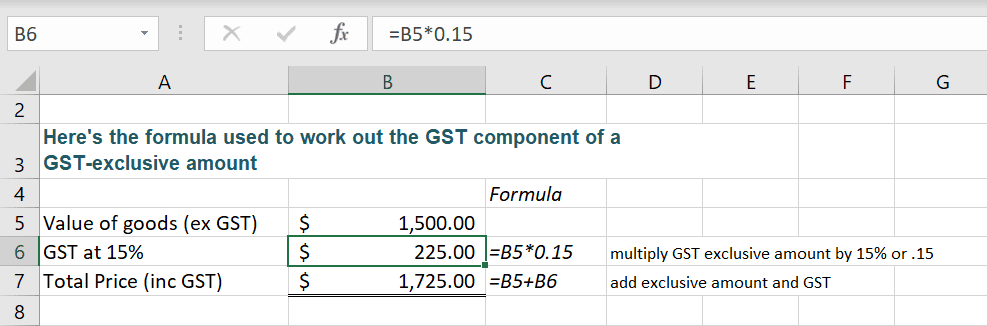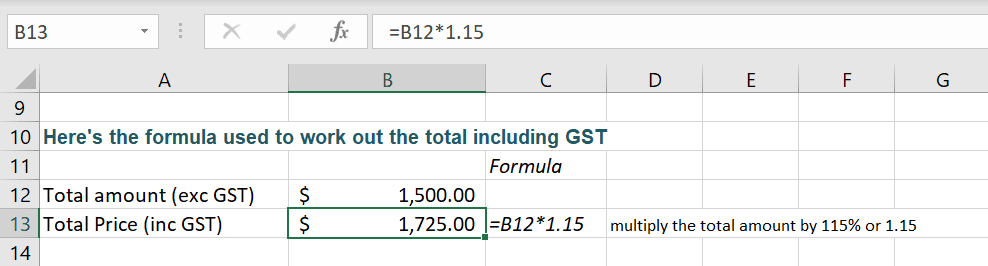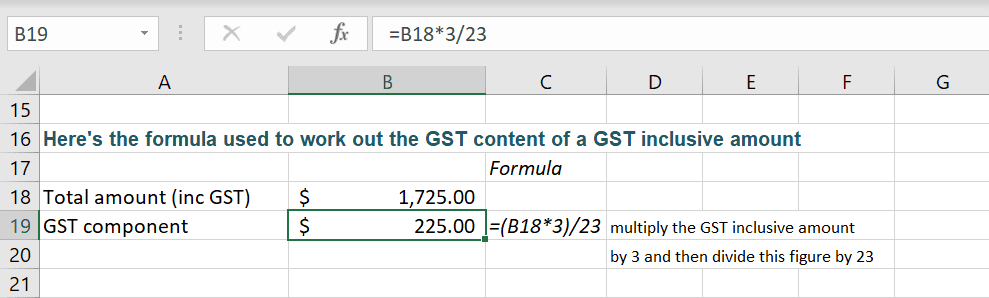Home » Our Blog » How to calculate GST at 15% using Excel formulas

# How to calculate GST at 15% using Excel formulasPost updated 18 October 2019 by Sharyn Baines

A common request I receive is to please explain how to calculate GST at 15% using Excel formulas.

You can find information on GST  (Goods and Services Tax) on the NZ Inland Revenue website. I’ve taken this a step further and put together a tutorial on how to put these calculations into Excel formulas.

Let’s start by calculating the GST component of a GST exclusive amount.  To do this you simply multiply the value, excluding GST by 15% or by 0.15.  To find the total including GST simply add the two values together.

In the example below B5 has been multiplied by 0.15, which is the same as 15%. You can type either value into the formula and Excel will give you the correct answer.

In cell B7 the GST exclusive amount held in B5 and the GST amount held in cell B6 have been added together to give the GST inclusive amount.## Formula for calculating the total including GST

There are times when you only want to calculate the Total figure including the GST. So let’s now have a look at calculating the total including GST.

This time we multiply the total by 115% or by 1.15.

In the example below cell B12, which is the figure without GST (exclusive figure) is being multiplied by 1.15 to calculate the Total including GST. The same result would have been found if you had used 115% instead of the 1.15.## Formula for finding the GST amount from a Total

Now let’s look at writing a formula to calculate the GST content of a GST inclusive amount.

This is extremely handy when you have a figure and you need to find how much GST is included in the Total figure. I use this a lot when I've purchased something for work and the receipt just shows the total figure without the GST figure.

This formula contains two parts.  First, multiply the GST inclusive amount by 3 and then divide this figure by 23.

In the example below the formula has been written as =B18*3/23

Note: if you like to use brackets, the formula will still calculate correctly if you use =(B18*3)/23Using these formulas you should now be able to quickly find and calculate GST.

In the video tutorial at the top of the page I take you step-by-step through each of the formulas listed above. Download the workbook and use it as your own GST calculator, or use it to follow along with the video.

The steps in the tutorial will work in Excel versions 2007, 2010, 2013 and 2016, 2019 and Excel for Office 365.

For more assistance with understanding GST (Goods and Services Tax) within New Zealand please visit the NZ Inland Revenue page for all things GST related.Sharyn is an expert trainer.  She became the first certified Microsoft® MOUS Authorised Instructor in New Zealand.

She is endorsed by Microsoft® as a qualified Microsoft® Office Specialist and has more than 20 years of experience in the training industry, developing and delivering technology training workshops. Her approach to taking the “techie-speak” out of technology training has placed her as a preferred supplier to many of New Zealand’s leading organisations.

## How to subtract in Excel

Let me help you learn how to subtract in Excel.   It’s simple when you’re shown how, but read to the end to make sure you understand what happens when you multiply and subtract, or divide and subtract in the same formula.   This is a tricky one, so please don’t skip over it!

## How to unhide columns in Excel

Need a hand to unhide columns in Excel?   There are different methods or unhiding available to you, depending on the version of Excel you are using.  I’ll take you through the method that will work on any version.

## Best Excel Keyboard Shortcuts for Beginners | Tips and Tricks

Learn the BEST Excel keyboard shortcuts. Perfect for Beginners and for those who want to save time and work smarter. It doesn’t matter if I’m delivering a Stage 1 or a Stage 3 Excel training course – when I’m demonstrating to the class I whip around the screen, format, select and modify and update using shortcut

## How to remove Excel formulas and keep the results

If you share Excel files outside of your organisation you’ll enjoy this post where I share with you how to remove Excel formulas and keep the results so that you can confidently send a file knowing you aren’t disclosing confidential information inside of your formulas.

## Move, Copy and Paste information in Excel

Learn how to move, copy and paste information in Excel. Once you have mastered Cut, Copy and Paste you will find yourself shifting data around easily and reusing copied data, saving you the need to recreate the same information again. All Windows applications have access to the Windows Clipboard. This is a special area used for

## Add colour, borders and alignment settings to Excel data

Learn how to add colour, borders and alignment settings to your Excel data to give your worksheet a more professional look and to make it easier for others to read.